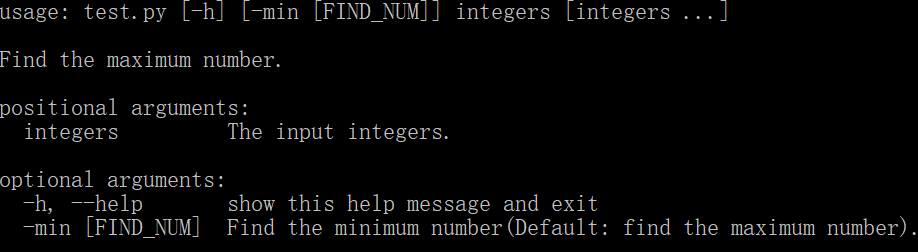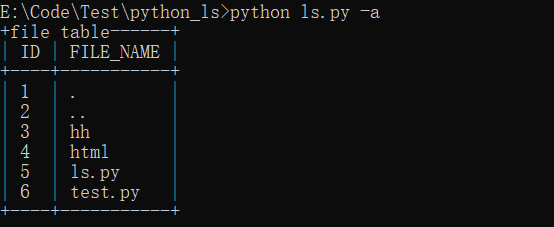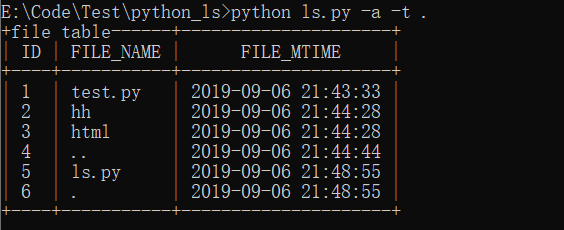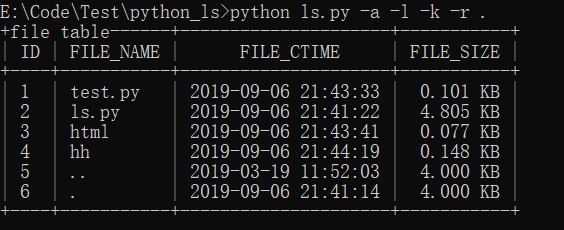# 使用Python代码实现Linux中的ls遍历目录命令的实例代码

更新时间：2019年09月07日 00:08:21   作者：TM0831我要评论

前几天在微信上看到这样一篇文章，链接为：https://www.jb51.net/it/692145.html，在这篇文章中，有这样一段话，吸引了我的注意：在 Linux 中 ls 是一个使用频率非常高的命令了，可选的参数也有很多， 算是一条不得不掌握的命令。Python 作为一门简单易学的语言，被很多人认为是不需要认真学的，或者只是随便调个库就行了，那可就真是小瞧 Python 了。那这次我就要试着用 Python 来实现一下 Linux 中的 ls 命令， 小小地证明下 Python 的不简单！

Linux ls 命令用于显示指定工作目录下的内容。语法如下：

ls [-alkrt] [name]

这里只列举了几个常用的参数，ls 命令的可选参数还是很多的，可以使用 man ls 来进行查看具体信息。这里列出的几个参数对应含义如下：

1）-a：显示所有文件及目录；

2）-l：除文件名称外，亦将文件大小、创建时间等信息列出；

3）-k：将文件大小以 KB 形式表示；

4）-r：将文件以相反次序排列；

5）-t：将文件以修改时间次序排列。

主要使用的模块是 argparse 和 os，其中 argparse 模块能设置和接收命令行参数，也就使得 Python 对命令行的操作变得简单，而 os 模块则用于文件操作，对 argparse 模块不熟悉的可以在这里查看官方文档。

既然要用 Python 实现 ls.py， 也就要在命令行中进行操作，比如 python ls.py -a 这样的命令，而对 Python 比较熟悉的人可能会想到使用 sys 模块来接收输入的命令，但使用 argparse 能让命令行操作变得更加简单！首先要导入模块并创建一个 ArgumentParser 对象，可以理解为一个解析器，然后就可以通过使用 add_argument() 方法为这个解析器添加参数了。示例如下：

```# test.py
import argparse
parser = argparse.ArgumentParser(description='Find the maximum number.')
parser.add_argument("integers", type=int, nargs="+", help="The input integers.")
parser.add_argument("-min", nargs="?", required=False, dest="find_num", default=max, const=min,
help="Find the minimum number(Default: find the maximum number).")

args = parser.parse_args()
print(args)
print(args.find_num(args.Nums))```> python test.py 1 3 5

Namespace(find_num=<built-in function max>, integers=[1, 3, 5])
5

> python test.py 1 3 5 -min

Namespace(find_num=<built-in function min>, integers=[1, 3, 5])

1

1）os.path.isdir(path)：若 path 是一个存在的目录，返回 True。

2）os.listdir(path)：返回一个列表，其中包括 path 对应的目录下的内容，不包含“.”和“..”，即使它们存在。

3）os.stat(path)：获取文件或文件描述符的状态，返回一个 stat_result 对象，其中包含了各种状态信息。

ls.py 中的主函数如下，主要功能为创建解析器，设置可选参数和位置参数，然后接收命令行参数信息，并根据输入的参数调用相应的方法，这里设置了一个 "-V" 参数用于显示版本信息，可以使用 "-V" 或者 "-Version" 进行查看。

``` def main():
"""
主函数，设置和接收命令行参数，并根据参数调用相应方法
:return:
"""
# 创建解析器
parse = argparse.ArgumentParser(description="Python_ls")
# 可选参数
parse.add_argument("-a", "-all", help="Show all files", action="store_true", required=False)
parse.add_argument("-l", "-long", help="View in long format", action="store_true", required=False)
parse.add_argument("-k", help="Expressed in bytes", action="store_true", required=False)
parse.add_argument("-r", "-reverse", help="In reverse order", action="store_true", required=False)
parse.add_argument("-t", help="Sort by modified time", action="store_true", required=False)
parse.add_argument("-V", "-Version", help="Get the version", action="store_true", required=False)
# 位置参数
parse.add_argument("path", type=str, help="The path", nargs="?")

# 命令行参数信息
data = vars(parse.parse_args())
assert type(data) == dict
if data["V"]:
print("Python_ls version: 1.0")
return
else:
check_arg(data)```

```def get_all(path):
"""
获取指定路径下的全部内容
:param path: 路径
:return:
"""
if os.path.isdir(path):
files = [".", ".."] + os.listdir(path)
return files
else:
print("No such file or directory")
exit()```

下面是 ls.py 运行后的部分结果截图。

首先是 python ls.py -a，这里并没有输入路径，就会使用默认路径即当前目录，如下图：最后是 python ls.py -a -l -k -r .，也是显示当前目录下的所有内容并按照创建名称排序，不过这次文件大小会以 KB 为单位来显示，如下图：```"""
Version: Python3.7
Author: OniOn
Site: http://www.cnblogs.com/TM0831/
Time: 2019/9/6 21:41
"""
import os
import time
import argparse
import terminaltables

def get(path):
"""
获取指定路径下的内容
:param path: 路径
:return:
"""
if os.path.isdir(path): # 判断是否是真实路径
files = os.listdir(path)
return files
else:
print("No such file or directory")
exit()

def get_all(path):
"""
获取指定路径下的全部内容
:param path: 路径
:return:
"""
if os.path.isdir(path):
files = [".", ".."] + os.listdir(path)
return files
else:
print("No such file or directory")
exit()

def check_arg(data):
"""
检查参数信息
:param data: 命令行参数（dict）
:return:
"""
assert type(data) == dict
if not data["path"]:
data["path"] = "."
# a参数
if data["a"]:
files = get_all(data["path"])
else:
files = get(data["path"])
# r参数
if data["r"]:
files = files[::-1]
# t参数
if data["t"]:
files = sorted(files, key=lambda x: os.stat(x).st_mtime)
for i in range(len(files)):
files[i] = [files[i], time.strftime("%Y-%m-%d %H:%M:%S", time.localtime(os.stat(files[i]).st_mtime))]
# l参数
if data["l"]:
result = []
for i in range(len(files)):
file = files[i] if data["t"] else files[i]
# 获取文件信息
file_info = os.stat(file)
# k参数
if data["k"]:
# 格式化时间，文件大小用KB表示
result.append([file, time.strftime("%Y-%m-%d %H:%M:%S", time.localtime(file_info.st_ctime)),
"%.3f KB" % (file_info.st_size / 1024)])
else:
# 格式化时间，文件大小用B表示
result.append([file, time.strftime("%Y-%m-%d %H:%M:%S", time.localtime(file_info.st_ctime)),
"{} Byte".format(file_info.st_size)])
if data["t"]:
for i in result:
i.append(time.strftime("%Y-%m-%d %H:%M:%S", time.localtime(os.stat(i).st_mtime)))

show_file(result, True, True) if data["t"] else show_file(result, True, False)
return
show_file(files, False, True) if data["t"] else show_file(files, False, False)

def show_file(files, has_l, has_t):
"""
格式化输出文件信息
:param files: 文件列表
:param has_l: 是否有l参数
:param has_t: 是否有t参数
:return:
"""
# 根据参数信息设置表头
if not has_l:
if not has_t:
table_data = [["ID", "FILE_NAME"]]
for i in range(len(files)):
table_data.append([i + 1, files[i]])
else:
table_data = [["ID", "FILE_NAME", "FILE_MTIME"]]
for i in range(len(files)):
table_data.append([i + 1] + files[i])
else:
if not has_t:
table_data = [["ID", "FILE_NAME", "FILE_CTIME", "FILE_SIZE"]]
else:
table_data = [["ID", "FILE_NAME", "FILE_CTIME", "FILE_SIZE", "FILE_MTIME"]]
for i in range(len(files)):
table_data.append([i + 1] + files[i])

# 创建AsciiTable对象
table = terminaltables.AsciiTable(table_data)
# 设置标题
table.title = "file table"
for i in range(len(table.column_widths)):
if i != 1:
# 居中显示
table.justify_columns[i] = "center"
print(table.table)

def main():
"""
主函数，设置和接收命令行参数，并根据参数调用相应方法
:return:
"""
# 创建解析器
parse = argparse.ArgumentParser(description="Python_ls")
# 可选参数
parse.add_argument("-a", "-all", help="Show all files", action="store_true", required=False)
parse.add_argument("-l", "-long", help="View in long format", action="store_true", required=False)
parse.add_argument("-k", help="Expressed in bytes", action="store_true", required=False)
parse.add_argument("-r", "-reverse", help="In reverse order", action="store_true", required=False)
parse.add_argument("-t", help="Sort by modified time", action="store_true", required=False)
parse.add_argument("-V", "-Version", help="Get the version", action="store_true", required=False)
# 位置参数
parse.add_argument("path", type=str, help="The path", nargs="?")

# 命令行参数信息
data = vars(parse.parse_args())
assert type(data) == dict
if data["V"]:
print("Python_ls version: 1.0")
return
else:
check_arg(data)

if __name__ == '__main__':
main()```

完整代码已上传到GitHub